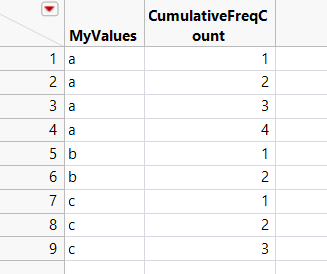Choose Language Hide Translation Bar
Highlighted

## how do a number sequence to unique values?

I have the  following Column1 and need to create a new column containing the sequential number of repetitions in Column1

 Column1 a a a b b c

The resulting table should look like this:

 Column1 Column2 a 1 a 2 a 3 b 1 b 2 c 1

Any suggestions how to code in in JSL? (or in column formula?) thank you!

1 ACCEPTED SOLUTION

Accepted Solutions
Highlighted

## Re: how do a number sequence to unique values?

Here is a column formula method:

``````// define values
Col1Vals = {"a","a","a","a","b","b","c","c","c"};

dt = New Table();

dt << New Column("MyValues",Character,Nominal,<< Set Values(Col1Vals));

// Create Cumulative Frequencies
dt << New Column("CumulativeFreqCount",Numeric,Continuous);
dt:CumulativeFreqCount << Set Each Value(
If(
Row() == 1, 1,
:MyValues == Lag( :MyValues, 1 ), Lag(:CumulativeFreqCount, 1 ) + 1,
1
)); // end set each value``````
4 REPLIES 4
Highlighted

## Re: how do a number sequence to unique values?

@rs ,

Assuming you have a reference to the data table - let us say dt :

``````dt1 = dt << Summary(
Group( :Column 1 ),
Freq( "None" ),
Weight( "None" )
)``````

If the data is not sorted (typically it should), then you can use the sort() on the resulting table

Best
Uday
Highlighted

## Re: how do a number sequence to unique values?

thank you.  I put the code into a JMP script file (see attachment) but the script does not run.  Any suggestions?

Would be easier to put the code in a column formula?

Highlighted

## Re: how do a number sequence to unique values?

Actually, I missed the part where you wanted to get a cumulative sum, rather just the sum.

I am pretty sure there is smarter and more efficient ways to do this. This will work though.

I am essentially creating the raw data as well, for you to test and play with

``````// define values
Col1Vals = {"a","a","a","a","b","b","c","c","c"};

dt = New Table();

dt << New Column("MyValues",Character,Nominal,<< Set Values(Col1Vals));

// Generate summary

dt1 = dt << Summary( Group( :MyValues ), Freq( "None" ), Weight( "None" ) );

UniqueItems = dt1:MyValues << Get values;
FrequencyOfItems = dt1 << Get As Matrix; Close(dt1,"No Save");

dt << New Column("CumulativeFreqCount",Numeric,Continuous);
for(i = 1, i <= N Items(UniqueItems),i++,
Rows = dt << Select Where(:MyValues == UniqueItems[i]) << Get Selected Rows;
ValsToAppend = Transpose(Index(1,FrequencyOfItems[i]));
dt[Rows,"CumulativeFreqCount"] = ValsToAppend;
dt << Clear Select;
); ``````Best
Uday
Highlighted

## Re: how do a number sequence to unique values?

Here is a column formula method:

``````// define values
Col1Vals = {"a","a","a","a","b","b","c","c","c"};

dt = New Table();

dt << New Column("MyValues",Character,Nominal,<< Set Values(Col1Vals));

// Create Cumulative Frequencies
dt << New Column("CumulativeFreqCount",Numeric,Continuous);
dt:CumulativeFreqCount << Set Each Value(
If(
Row() == 1, 1,
:MyValues == Lag( :MyValues, 1 ), Lag(:CumulativeFreqCount, 1 ) + 1,
1
)); // end set each value``````
Article Labels

There are no labels assigned to this post.